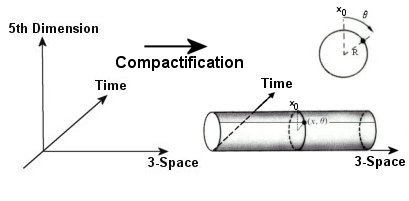In History by Brian Koberlein1 Comment

We usually think of efforts to unify Einstein’s theory of gravity with other forces as a more modern trend. Models such as string theory and loop quantum gravity are seen as modern ideas. But in fact, as soon as Einstein presented his model there were efforts to unify it with the other known force at the time, electromagnetism.

In 1919, the same year that Eddington confirmed Einstein’s theory. Theodor Kaluza proposed a generalization of Einstein’s model that had five dimensions, rather than the four (3 space + time) of general relativity. Although the general five-dimensional model was quite complex, Kaluza found that if the fifth dimension was considered to be somewhat independent of the others (known as the cylinder condition), then something interesting happened. The general equations could be broken into three parts: a 4-dimensional model that was Einstein’s equations for gravity, a separate set of equations that matched Maxwell’s equations for electromagnetism, and a scalar field known as the radion. In other words, the 5-dimensional theory seemed to unify gravity and electromagnetism into a single model.

In 1926 Oskar Klein applied quantum theory (as it was understood at the time) to Kaluza’s model. He proposed that the extra spatial dimension was “compact,” which would explain the cylinder condition. Klein then found that the scale of this compact dimension was connected to the quantization of charge. Soon this model came to be known as the Kaluza-Klein model.

At the time, the strong and weak nuclear forces were unknown, so the Kaluza-Klein model held promise as a single unified theory to describe all of known physics. But it didn’t turn out to be the case. There are several reasons for that. One is that while the simplifed “cylinder” form of the model clearly gave rise to general relativity and electromagnetism, the general model was deeply complex and difficult to understand. Another is that as quantum theory became more robust, the Kaluza-Klein model was found difficult to quantize. It wasn’t until the 1940s that the classical version was finally understood, much less a fully quantum model. Finally, the discovery of the strong and weak nuclear forces meant that the Kaluza-Klein model was a partial theory at best. As the standard model of particle physics was developed (unifying electromagnetism with the strong and weak forces), the popularity of the Kaluza-Klein model waned.

In many ways, the Kaluza-Klein model was the string theory of its day. It had some early successes, followed by a deep complexity that made progress difficult and slow.  In fact aspects of the Kaluza-Klein model such as higher dimensions and compact manifolds also appear in string theory and other modern models.

Although not as popular as it once was, there is still some interest in the Kaluza-Klein model. The radion scalar field has some similarities to the Higgs field, and the radion could affect the way the Higgs interacts with photons. So some researchers hope that data from the Large Hadron Collider might show evidence of such an interaction.

But even if such evidence is never found, the Kaluza-Klein model is an interesting bit of theoretical physics history. It showed that gravity and electromagnetism do share a deep mathematical connection, and it introduced theoretical ideas that are central to modern models.

1.